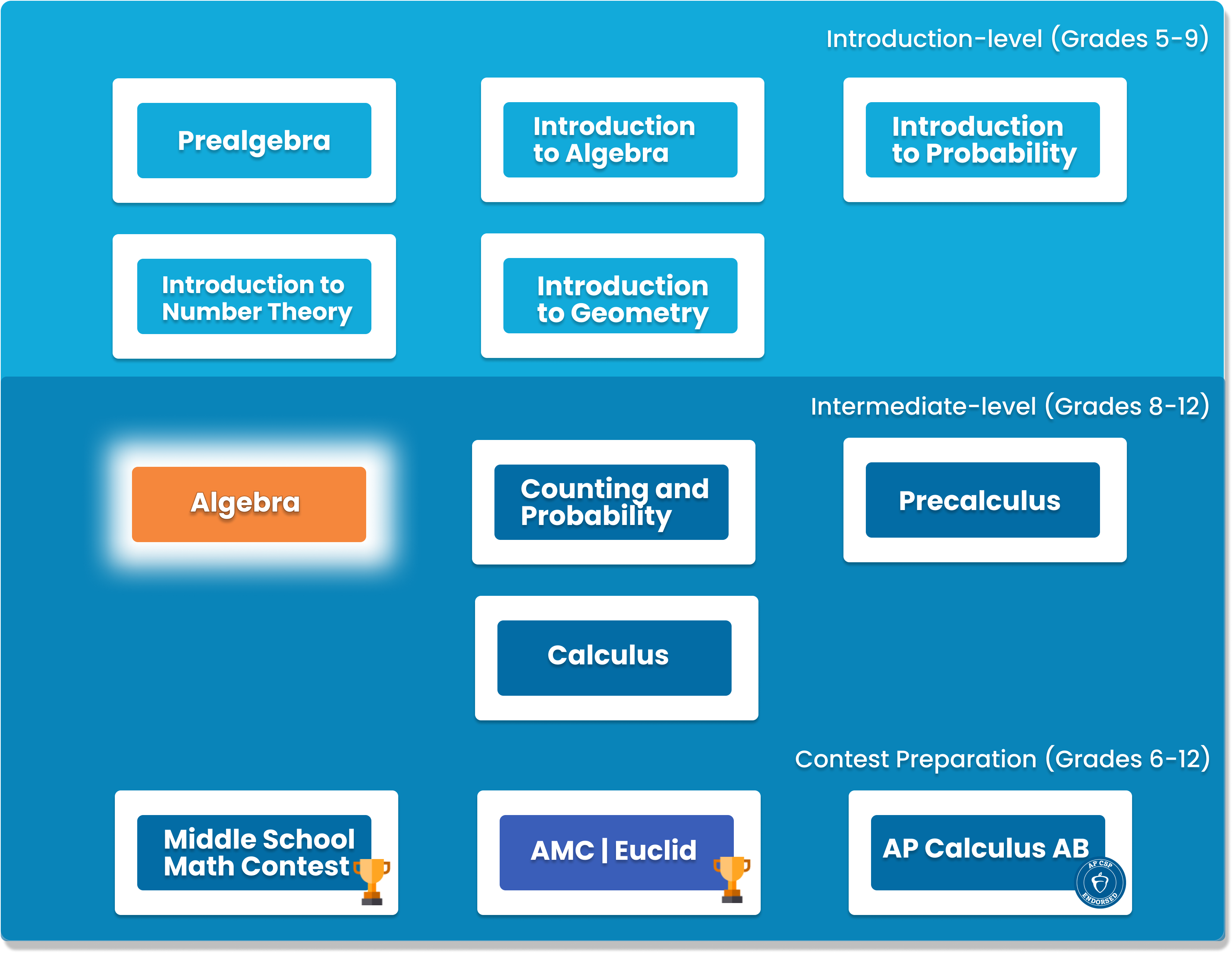# Algebra

This class will build on concepts learned in the Pre-Calculus and Introduction to Calculus courses. Students will learn advanced concepts that include polynomials, inequality, radicals, and functions. This class is designed to expand on the Algebra and Pre-Calculus curriculum to introduce students to the problem solving strategies required for success on the AMC and AIME competitions.##### Who is this course for
This course is for students who have completed our Introduction to Algebra course.
This course typically takes 3-6 months to complete. This depends on the student's experience and how fast they can master the concepts and the knowledge. Our instructors move at the pace of the student, it may take extra time for some students to reinforce what they have learned.
##### Curriculum
###### Chapter 1: Basic Techniques for Solving EquationsSolving system of equations by substitution and elimination
###### Chapter 2: Functions ReviewDefinition of functions, graph of functions, composition, and inverse functions
###### Chapter 3: Complex NumbersArithmetics of complex numbers, complex planeFactoring, Vieta’s formulae, discriminant, quadratic inequalities
###### Chapter 5: ConicsParabolas, Circles, Ellipses, Hyperbolas
###### Chapter 6: Polynomial DivisionDivision, synthetic division, remainder theorem
###### Chapter 7: Polynomial Roots IFactor Theorem, Fundamental Theorem of Algebra
###### Chapter 8: Polynomial Roots IIIrrational and complex roots, Vieta’s Formula
###### Chapter 9: Factoring Multivariable PolynomialsGrouping, sums and differences of powers
###### Chapter 10: Sequences and seriesArithmetic series and sequences, geometric series and sequences, summation and product notation
###### Chapter 11: Identities, Manipulations, and InductionBrute Force, Induction, Binomial Theorem
###### Chapter 12: InequalitiesTrivial and AM-GM inequality, Cauchy Schwarz inequality
###### Chapter 13: Exponents and LogarithmsExponential Functions, Properties of LogarithmWorking with expressions with radicals, conjugates
###### Chapter 15: Special Classes of FunctionsRational Function, even and odd functions, monotonic functions
###### Chapter 16: Piecewise Defined FunctionsPiecewise functions, absolute value, floor and ceiling
###### Chapter 17: More Sequences and seriesRecursive Sequences, Telescoping series
###### Chapter 18: More InequalitiesRearrangement Inequality
###### Chapter 19: Functional EquationsSolving functional equations with substitution, separation
###### Chapter 20: Some advanced strategiesSolving problems by exploiting the symmetry in the problem, substitution, using undetermined coefficients

### You Might Also Be Interested In Our Elective Courses##### Python Level 1
AGES 10 - 15

Designed for beginners, this level is designed to teach the basic fundamentals and design principles of Python, with the help of Turtle graphics and PyGame. This includes: variables, conditional statements, loop basics, and functions.

These concepts are transferable to any other programming language. Throughout the way, students will create projects in order to apply the concepts they have learned, and to solidify their knowledge.##### Python Level 2
AGES 10 - 15

Level 2 dives deeper into the basics of Python for a more thorough understanding and introduces advanced topics. Students will build on knowledge from Level 1 and work with data structures, advanced loops, algorithms, and object-oriented programming, and create games based on what they learn.

Students will complete the course with a solid understanding of Python fundamentals.##### Java Level 1
AGES 10 - 15

This beginner-friendly course serves as an introduction to the Java programming language. Students will learn the fundamentals of Java along with core computer science concepts. Concepts covered in this course include Java syntax, types and identifiers, operators, if statements, loops, arrays and more.

Students will apply these concepts to create fun games such as Tic-Tac-Toe.